Ideas to Review for AP 2 Test
Below are a series of simulated situations used to illustrate major ideas in physics. Next to each link I give you a few things to consider as you explore the environment that was recreated in the program.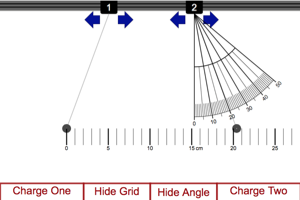Coulomb's Law Lab Adjust the different factors and notice how the angle of displacement from the vertical changes. This angle is a result of the force on the charge and can be used as a gauge as to how much force is on the charge.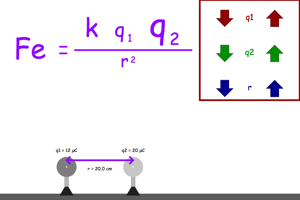Force Electric Mini Lab Adjust the different factors and note their affect on the force between the two charges.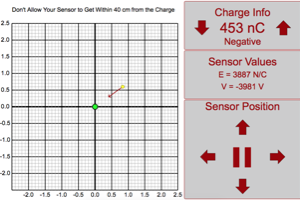Space Around Charge Lab The yellow circle is a sensor that can determine the electric potential and the electric field. Move the sensor around and notice the direction and strength of the electric field. Change the charge from negative to positive and back again and notice the direction of the electric field. Put your sensor right on the y-axis about 50 cm from the charge. Notice the strength of the field and the value of the electric potential. Double the distance between the sensor and the charge. Notice the changes that take place.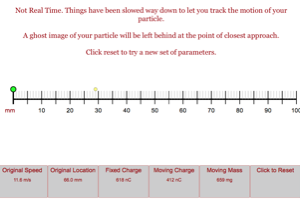Closest Approach Between Particles Lab Fire your charged particle at fixed charge. Notice how close the moving charge gets to the fixed charge. Adjust the parameters and fire again. Notice how different factors affect the distance of closest approach. Think of this in terms of energy. How is the original energy of the particle turning into energy of the system?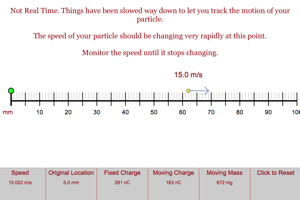Velocity from Repulsion Lab Bring your moveable charge as close to the fixed charge as possible. Release the charge and watch as it is repelled away from the fixed charge. When does the most rapid change in speed occur? Why does the speed eventually seem to plateau? Think about how the energy of the system become kinetic energy of the moving particle.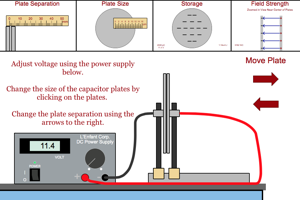Capacitor Properties Clicking on the "Storage" tab will let you see how much energy and charge are stored by the capacitor. Clicking on the field strength tab will allow you to see the strength and direction of the electric field in the region between the plates of the capacitor. Change the different parameters of the capacitor and the power supply and see how the field changes and how the amount of energy and charge being stored by the capacitor changes.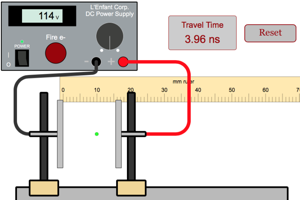Accelerating Electrons Use your capacitor to accelerate electrons between the plates of the capacitor. You can use the distance traveled, the time of travel and the initial speed of the electron to find the acceleration, final speed or force on the electron. How do different changeable factors affect these values? Think of the situation in terms of energy.Ohm's Law with Analog Meters Notice how current is affected by voltage and resistance.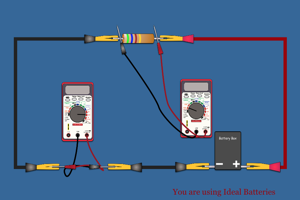Simple Circuits Notice how the current is affected by the voltage of the batteries and the resistance of the resistor. Click on the battery box to get the voltage (each battery adds another 1.5 V). Click on the resistor to get its colors and hence its resistance.Series Circuits What is true of the current in this series circuit? What is true of the voltage lost in the resistors compared to the total voltage of the battery pack? Click on the battery box to get the voltage (each battery adds another 1.5 V).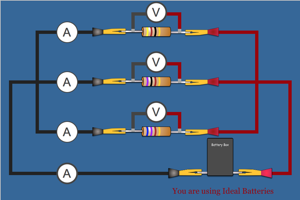Parallel Circuits What is true of the current in this parallel circuit? What is true of the voltage lost in the resistors compared to the total voltage of the battery pack? Click on the battery box to get the voltage (each battery adds another 1.5 V).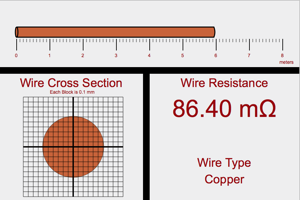Resistance of Wire What are the three factors that determine the resistance of a wire? How does each factor contribute to determining the resistance of the wire?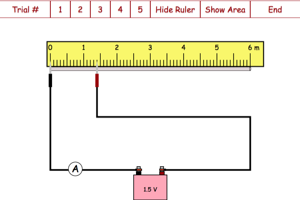Resistance of Wire based on Ohm's Law This lab is designed to have students work through a procedure that will allow them to determine the resistivity of a wire based on the amount of current going through different lengths of wire.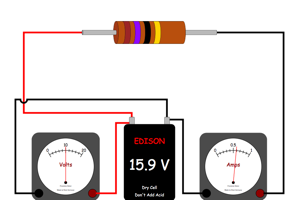Internal Resistance of Battery Work with resistors with a really low resistance (black as the third band). Notice how the terminal voltage of the battery drops as you lower the resistance of the resistor. Take 5 data points where you record the current from the battery and the terminal voltage of the battery. Make a quick plot to notice the trend between the current from the battery and the terminal voltage of the battery. Use your graph to find the maximum current, the electrochemical potential of the battery and the internal resistance of the battery.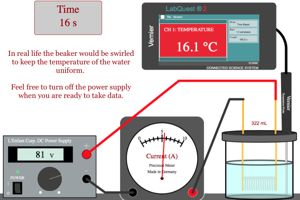Electrical Equivalent of Heat Record the original temperature and mass of your water. Turn on the power supply for 60 seconds. Record the voltage and current from the power supply. At the end of the 60 s turn off the power supply and record the new temperature of the water. Use the voltage and current from the power supply to get the energy delivered to the water in the 1 minute of collecting data. Calculate the thermal energy added to the water. In real life, what are some things that might make these two energies not quite equal?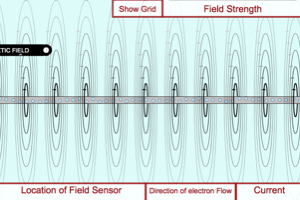Magnetic Field from Wire Notice the shape of the magnetic field around the wire. Change the direction of the current flow around the wire and notice the change to the direction of the magnetic field. Change the location of the field sensor and notice the strength of the magnetic field. Change the amount of current moving through the wire and notice the change it causes to the strength of the magnetic field. Turn on the 2 dimensional representation of the magnetic field. Make sure you understand how the 2d representation is used to represent the 3d field.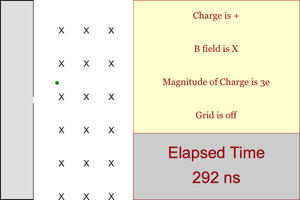Charge in Magnetic Field Fire the charge into the magnetic field and notice the size of the arc it follows. Change all the parameters and make sure you understand how each parameter affects the arcs size and direction.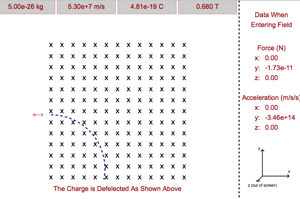Charge Moving Through Magnetic Field Basic Make sure you know how to calculate the force magnetism on a charge fired through a magnetic field. Once you know the force, you should be able to calculate the acceleration of the charge, and finally you should know how to predict the size of the arc followed by the charge as it moves through the magnetic field.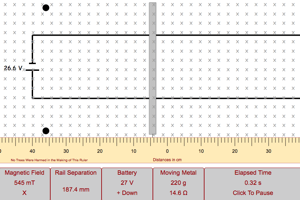Rail Gun Lab Send a current through the metal bar that is resting on a set of conducting rails. Make sure you understand why the bar moves and why it goes in the direction that you see it travel. Change the different parameters and make sure you know how each parameter affects the acceleration of the bar.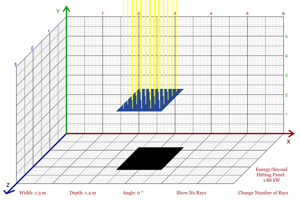Solar Flux Lab Change different parameters to see which things affect the amount of solar flux that is hitting the solar panel. The main thing to get from this lab is an idea of what is meant by the term flux.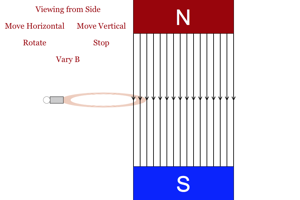Magnetic Induction Make changes and watch the light. When the light is on that shows there is an induced current in the coil. Make sure you understand each thing that causes the light to be on and why some things don't cause the light to come on. From this make sure you understand how magnetic flux influences whether the light will be on or off.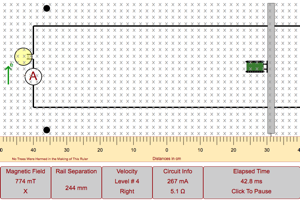Induced Current Lab In this simulation you should focus on the quantitative relationships that determine the amount of current that is produced by the changing flux. Change each of your variables and see how they influence the amount of current that flows in your circuit.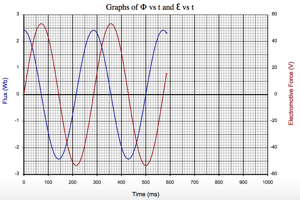Spinning Coil in Magnetic Field with Graph Lab Look at the graphs for flux vs. time and electromotive force vs. time for a coil spinning in a magnetic field. Change each factor and see how it affects the electromotive force. Make sure you can read the graphs to determine period, frequency, maximum electromotive force and the rms of the electromotive force.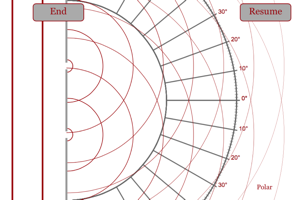Diffraction of Light Make sure you understand the diffraction that is occurring in this program. Make sure you understand the interference that is occurring in this program. Examine the different factors affecting the nature of the diffraction pattern on the wall to the right of the diffraction grating.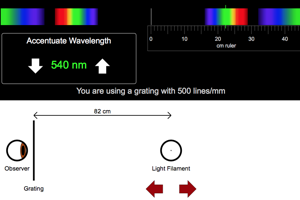Electromagnetic Spectrum with Diffraction Grating The white light is being viewed through a diffraction grating and you are to look at how changing different parameters can lead to the colors appearing in different locations. Notice how there are higher order patterns that are occurring beyond the location of the first order fringes.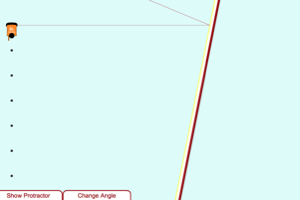The Way a Mirror Works Lab (Plane Mirror) The hockey pucks bouncing off the boards is a mechanical analogy to light bouncing off a mirror. Angle the boards to the direction of the incoming pucks and then pull up the protractor. Notice the relationship between the angle of the incoming pucks and the angle of the reflected pucks.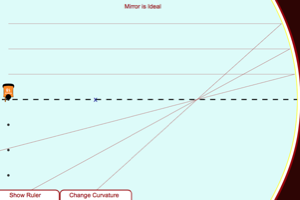The Way a Mirror Works Lab The hockey pucks bouncing off the boards is a mechanical analogy to light bouncing off a mirror. The boards are curved to form a surface like a converging mirror. The "X" on the ice marks the center of curvature. Notice how all the reflected paths pass through a single spot on the ice. Notice where this spot is located relative to the center of curvature of the boards. Change the curvature of the boards and see how the location of the point of intersection changes.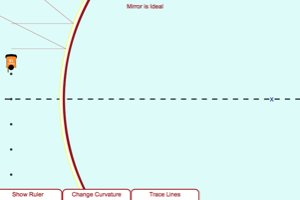The Way a Mirror Works 2 Lab The hockey pucks bouncing off the boards is a mechanical analogy to light bouncing off a mirror. The boards are curved to form a surface like a diverging mirror. The "X" on the ice marks the center of curvature. Notice how all the reflected paths trace back to a single spot on the ice. Notice where this spot is located relative to the center of curvature of the boards. Change the curvature of the boards and see how the location of the point of intersection changes.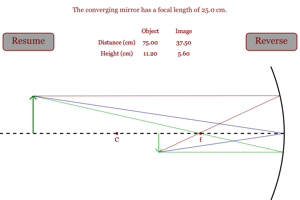Converging Mirror Lab Watch how the image created by a converging mirror changes as the object changes its location relative to the mirror. Note the relationship between the height of the image and the location of the image based on where the object is placed relative to the focal point and center of curvature for the mirror. When is the image the same height as the object?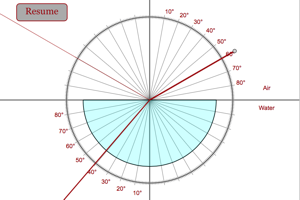Refraction of Light When the program opens, pause it and then zero the angle. You will see light coming out of the little gray circle, travel through the air and then enter into a semicircular tank of water. No change in path should occur when the light moves from air to water. Now resume the simulation so that the light enters at greater and greater angles with respect to the normal line. Notice how the angle of the refracted light is less than the angle of the incident light. Now reverse the location of the light so that it moves from water to air. Now you should see the angle of refraction larger than the angle of incidence. Once the angle of incidence gets too large, the light can no longer escape the water.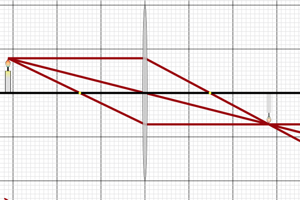Images from Lenses Notice the path of the three primary rays of light leaving the object. Where they meet back up after passing through the lens is where the image will form. Notice how the image is different from the original object. Change the location of the object and the focal length of the lens. How do these changes affect the image?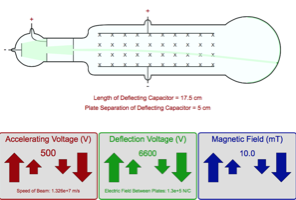Thompson Cathode Ray Tube Lab Adjust the strength of the magnetic field or the electric field to change the amount of deflection of the beam. What conditions must exist for the beam to travel in a perfectly straight line when in between the plates of the capacitor?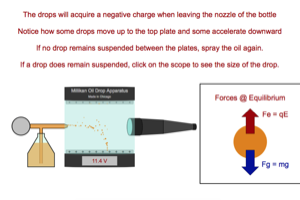Millikan Oil Drop Lab Follow the directions to determine the charge on the oil drop. Do this a few times and you should see that the drops always have a charge that is an integer multiple of the charge of an electron. This famous experiment showed that charges are quantized and are always some multiple of 1.602e-19 C.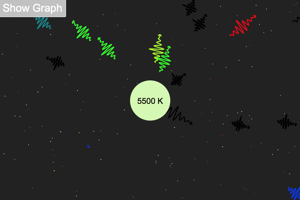Radiant Emission of Light Watch the emission of light by stars of different temperatures. Look at the graphs to see the relative intensity of each different wavelength of light. Notice the change in the total amount of light emitted per second as the temperature of the star increases.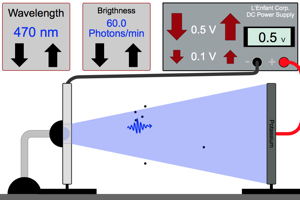Photoelectric Effect Lab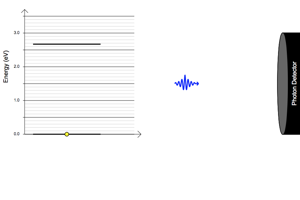Frequency from Energy Gap Allow the electron to fall from one energy level to another. Notice the frequency of the photon that is emitted. Run a few different trials to see how the gap in energy levels affects the frequency of the light emitted.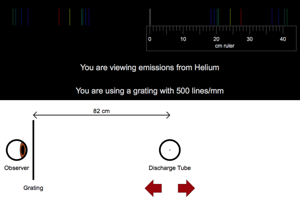Emission Spectra with Diffraction Grating Just take a look at the spectra of a few different elements that are giving off light. Realize that each unique color that you see is a result of an energy level different in the atoms of that element.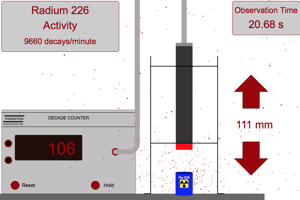Radiation Detection Lab Do a few trials to see how the distance from a radioactive source affects the amount of decays that are detected by the detector.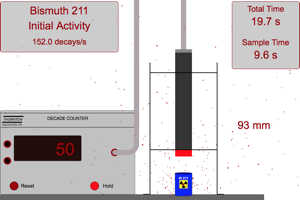Half-Life Lab Collect 10 seconds worth of data at 40 second intervals to see how the number of decays changes as time goes on.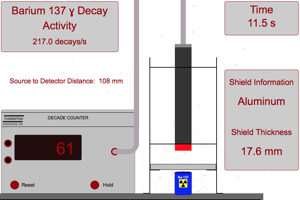Shielding Lab Place different shields between your detector and the radioactive source. How does the thickness of the shield, what the shield is made of, and the type of decay all affect the number of counts your detector registers?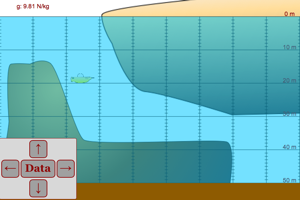Deep Sea Diver Take a few pressure measurements at a few different depths. Plot out the total pressure versus depth. What is the significance of the y-intercept of your graph? What is the value of your slope determined by?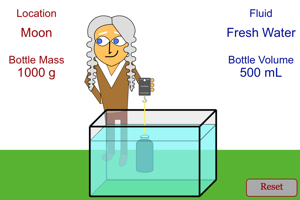Buoyancy Lab The force of buoyancy can be found by looking at the change in tension that occurs as the bottle is moved from air into the fluid. See how each of the factors changes the force of buoyancy on the bottle.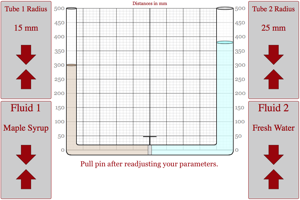Fluids in a U-Tube Lab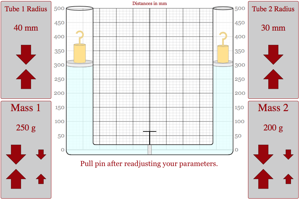Pascal's Principle Lab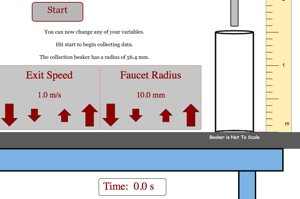Flow Rate Lab Play around with the program to make sure you know how to determine the flow rate from a hose. Then remind yourself of the factors that determine the flow rate from a faucet.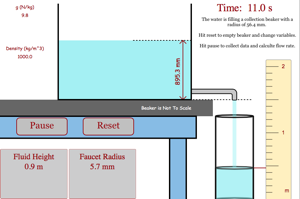Flow Rate From Beverage Dispenser Lab Based on what you know about flow rate, play around to remind yourself of the formula that links flow rate to properties of the faucet, fluid, planet and height.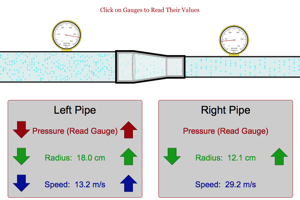Bernoulli Lab This environment has been created to allow students to look at how the size of pipes affects the speed and pressure of the fluid moving through the pipe.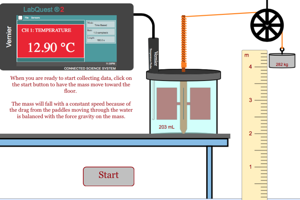Mechanical Equivalent of Heat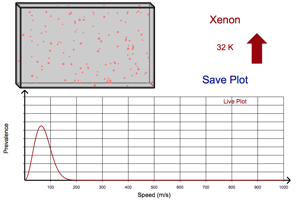Maxwell Distribution Lab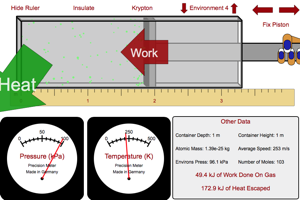Gas in Box Lab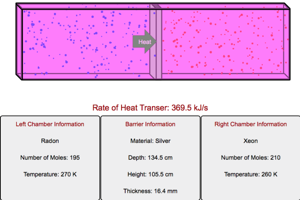Heat Transfer Lab